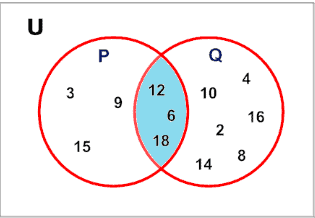# Python Set intersection_update() Method

Before going through an intersection_update() method, we should know what an intersection is. It refers to the common elements of two given sets. This means if there are two sets and they have some common elements then those common elements are known as the intersection of both the element.## Python Set intersection_update()

The set intersection_update() is a built-in Python method that is similar to the intersection() method with a difference that in the intersection() method, the caller set remains unchanged, but in the intersection_update() method, the caller set is updated with the result value. This means the caller set is updated with the intersection value of two sets.

Python intersection_update() method updates the set calling intersection_update() method with the intersection of sets. The intersection of two or more sets is the set of items that are common to all sets. To learn more, visit the Python set Intersection.

## How intersection_update() works in Python

Let’s say we have two sets A and B, then A.intersection_update(B) operation updates set A with common elements in set A and B. For example, A=set([1,2,3]) and B=set([4,2,3]) now after taking A.intersection_update(B), value of set A will be [2,3].

### Syntax

```First_Set.intersection_update(Second_Set)
```

Here, First_Set is called a caller set, which is updated with the result value, and Second_Set is the set with which we find an intersection.

### Return Value

The intersection_update() method returns None. But it updates the value of the caller set with the result value.

See the following code example.

```# app.py

# Declaring two sets
# Even nums between 2 and 10
set1 = {2, 4, 6, 8, 10}
# Multiple of 3 between 1 to 10
set2 = {3, 6, 9}

# priting both the sets
print("Set1 is: ", set1)
print("Set2 is : ", set2)
# Now we will find intersection of these two sets
result = set1.intersection_update(set2)
print("Result value is: ", result)
print("Value of set1 is: ", set1)
print("Value of set2 is : ", set2)
```

#### Output

```Set1 is:  {2, 4, 6, 8, 10}
Set2 is :  {9, 3, 6}
Result value is:  None
Value of set1 is:  {6}
Value of set2 is :  {9, 3, 6}```

From the output, we can see that, before calling the intersection_update() method, the value of set1 was different, but after calling the intersection_update() method, the value is changed. This is because, as we learned, this method updates the value of the caller set ( which is set1 ) with the result value.

Also, we can see that the value of the result is None as this method returns None and set2 value is unchanged.

## Python intersection_update() with Two Parameters

You can pass two parameters to the intersection_update() method.

See the following code.

```# app.py

A = {1, 2, 3, 4}
B = {2, 3, 4, 5, 6}
C = {4, 5, 6, 7, 8}

result = C.intersection_update(B, A)

print('result =', result)
print('C =', C)
print('B =', B)
print('A =', A)
```

#### Output

```python3 app.py
result = None
C = {4}
B = {2, 3, 4, 5, 6}
A = {1, 2, 3, 4}```

In the above example, we have three Sets A, B, and C. Here, we are passing more than one parameter to the intersection_update() method.

To find the intersection between more than two sets, we can pass the additional sets in the intersection_update() method, and we got the output.

## Equivalent Operator(&=) in Python

You can achieve the same result of the intersection_update() method by using the &= augmented assignment operator.

See the following code.

```# app.py

A = {1, 2, 3, 4}
B = {2, 3, 4, 5, 6}

A &= B
print('A =', A)
```

#### Output

```python3 app.py
A = {2, 3, 4}```

That’s it for this tutorial.